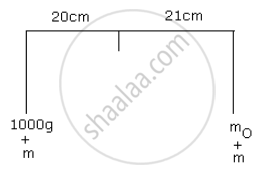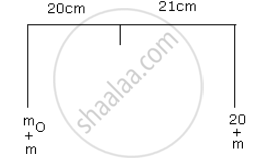Advertisement Remove all ads

# The Arms of a Beam Balance Are 20 Cm and 21 Cm, but the Pans Are of Equal Weight. by the Method of Double Weighing the Weights Are Found to Be 1000 G and 20 G. Find the Actual Weight of the Body - Physics

Numerical

The arms of a beam balance are 20 cm and 21 cm, but the pans are of equal weight. By the method of double weighing the weights are found to be 1000 g and 20 g. Find the actual weight of the body

Advertisement Remove all ads

#### Solutionm  = mass of each pan

m0 = actual mass of the body

(100 + m)20 = (m + m0) 21Dividing the two equations

21/20 = ((100 + "m")20)/((20 + "m") 21)

1.1 (20 + m) = (1000 + m)

22 + 1.1m = 1000 + m

0.1m = 978

m = 9780g

Is there an error in this question or solution?
Advertisement Remove all ads

#### APPEARS IN

Frank ICSE Class 10 Physics Part 2
Chapter 1 Force, Work, Energy and Power
Exercise | Q 5.3 | Page 61
Advertisement Remove all ads
Advertisement Remove all ads
Share
Notifications

View all notifications

Forgot password?
Course